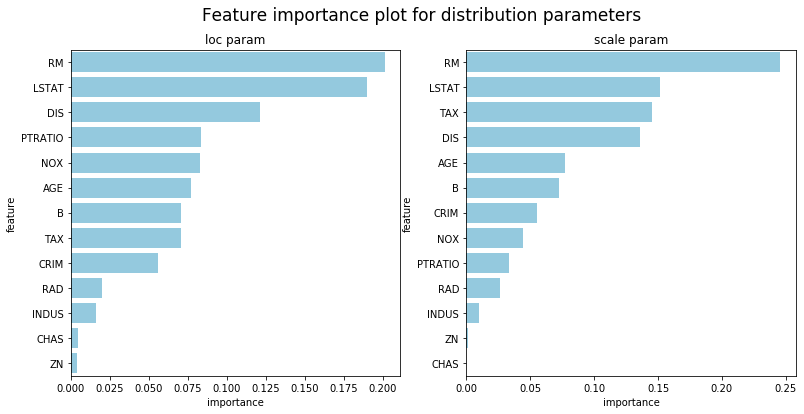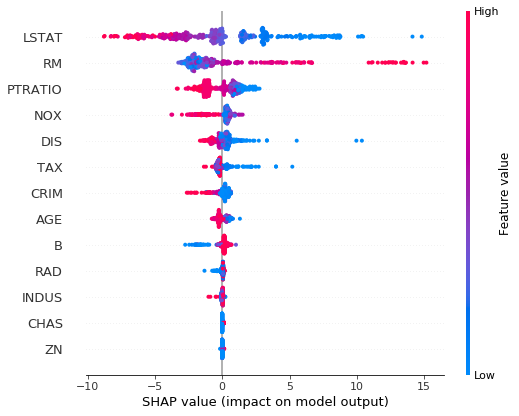Model Interpretation
from ngboost import NGBClassifier, NGBRegressor
from ngboost.distns import k_categorical, Normal
from ngboost.scores import LogScore

from sklearn.model_selection import train_test_split

X_reg_train, X_reg_test, Y_reg_train, Y_reg_test = train_test_split(X, Y, test_size=0.2)

y[0:15] = 2 # artificially make this a 3-class problem instead of a 2-class problem
X_cls_train, X_cls_test, Y_cls_train, Y_cls_test  = train_test_split(X, y, test_size=0.2)


We caution users against trying to interpret too much from any machine learning model, but NGBoost does provide methods to interpret models fit with regression tree base learners. Since each parameter in the distribution is fit by a separate sequence of learners, there will be multiple model interpretation results, one for each parameter. The default distribution used is Normal so the following example shows results for the loc and scale parameters.

## Feature Importance

ngb = NGBRegressor(verbose=False).fit(X_reg_train, Y_reg_train)

## Feature importance for loc trees
feature_importance_loc = ngb.feature_importances_

## Feature importance for scale trees
feature_importance_scale = ngb.feature_importances_

import pandas as pd
import matplotlib.pyplot as plt
import seaborn as sns

'importance':feature_importance_loc})\
.sort_values('importance',ascending=False)
'importance':feature_importance_scale})\
.sort_values('importance',ascending=False)

fig, (ax1, ax2) = plt.subplots(1, 2, figsize=(13,6))
fig.suptitle("Feature importance plot for distribution parameters", fontsize=17)
sns.barplot(x='importance',y='feature',ax=ax1,data=df_loc, color="skyblue").set_title('loc param')
sns.barplot(x='importance',y='feature',ax=ax2,data=df_scale, color="skyblue").set_title('scale param')

Text(0.5, 1.0, 'scale param')## SHAP

import shap
shap.initjs()

## SHAP plot for loc trees
explainer = shap.TreeExplainer(ngb, model_output=0) # use model_output = 1 for scale trees
shap_values = explainer.shap_values(X_reg_train)Setting feature_perturbation = "tree_path_dependent" because no background data was given.